# How to teach square of 5

in Educationlast month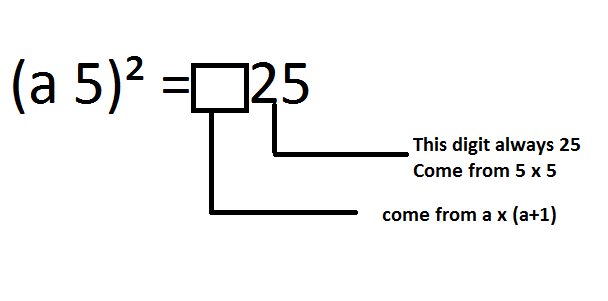Hi all friends...

When students study one of the subjects they hate is Mathematics. Many children say Math is boring, difficult, and dizzying. Mathematics only creates problems in learning.
In fact, Mathematics is a lesson that will guide everyone in life. By studying Mathematics, we will be directed to become a person who is precise, careful and full of calculations in making decisions.
Because many children have difficulty learning Mathematics, it is the duty of parents to help overcome these difficulties. It's good for parents to know how to learn Mathematics correctly and practically so that it makes learning Mathematics more fun.

### The square of a multi-digit number with a unit number of 5

Well, this time I will share the calculation of the square. A square is a product of the same number.
That is, the square can be formulated as:For example, the square of 5 is 5 x 5 and the result is 25.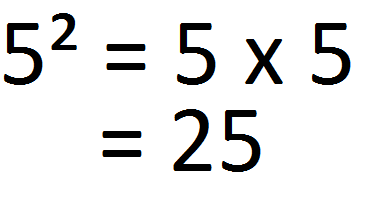In the multiplication of the same two numbers we can get interesting things. In an interesting way, we will be able to make children feel happy knowing that Mathematics is fun. At least we can use it as an intermezo material when children feel bored with learning.
The square of the number with the last digit 5 ​​can be found in several ways. It can be the long way or the short way.
The long way is to multiply normally. What is commonly done is to multiply the stack down. While the quick way can be formulated withIn the figure above, the result of the square can be divided into two parts. The first part is represented by a square and the second part is the number 25. In the box, it is filled in by multiplying the first digit by the first digit plus 1.

Let's look at the following example.
Example 1.
The square of 55 is...
We can do this by multiplying 55 by 55 as in the image below.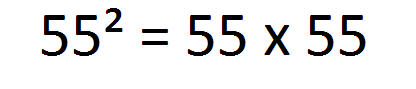If we use the long way then we can do it by multiplying stacking down.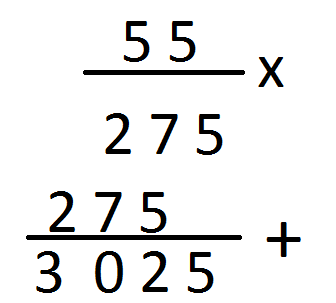The result of calculating the square of 55 is 3025.
Well, now let's use the quick way.
The number that is squared has two digits. The first digit is five and the second digit is also 5.
The method:
Multiply the first digit by the first digit plus 1 so that it becomes a multiplication of 5 and 6 . Add 25 after the multiplication result.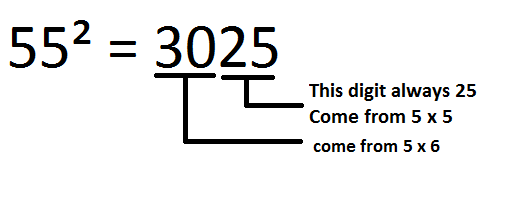Example 2.
The square of 75 is...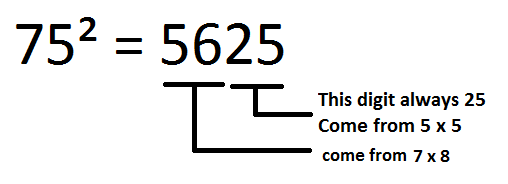With these two examples we can determine the square of the other number as follows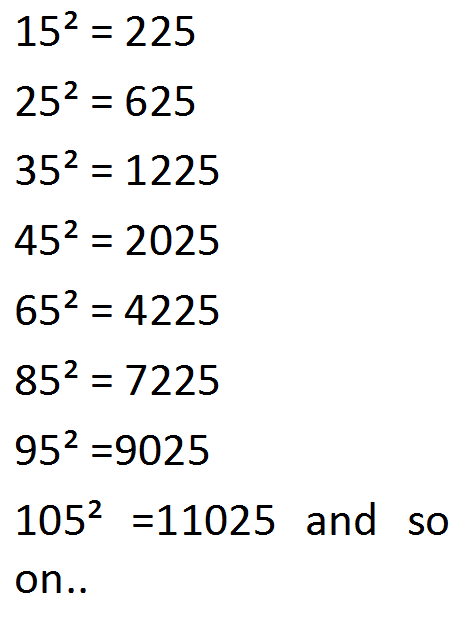That's how to teach the square of numbers with unit digits 5. In this way, it is hoped that children will feel happy learning mathematics.
Thus my writing this time may be useful.

Best regard from Indonesia.
@rokhani

# Thank you for stopping by and reading my post. I hope you enjoy it. Upvote, comment, reblog and other support are highly appreciated.

Sort:

Your content has been voted as a part of Encouragement program. Keep up the good work!

Use Ecency daily to boost your growth on platform!

Support Ecency
Vote for Proposal
Delegate HP and earn more